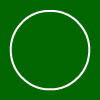# Circle - Area and Circumference

These are the formulas for calculating circles:
Circumference = 2 * pi * radius

## What is a circle?A circle is a shape with all points at the boundary having the same distance to the centre.
The following formulas are used for circle calculations. The area of a circle is A = pi multiplied with r▓ and the circumference is U = 2 multiplied with pi multiplied with r , in which pi is the circle constant (approximately 3,14).

## How are the values calculated?

Enter a few values into the input fields and press äcalculateō. Then you will see it.

## What interesting lines exists for a circle?

First of all, there are lines that don't intersect the circle that are called passants. Lines that intersect the circle twice are called secants. More interesenting are the the lines that intersect the circle in exactly one point. They are called tangents.

## What are the names of the cirlce parts?

Move the cursor over the below-mentioned word. The corresponding circle part will be colour-marked.Radius Diameter Circumference Area of a circle